### Home > PCT > Chapter Ch9 > Lesson 9.3.2 > Problem9-114

9-114.
1. A rectangular corral is to be built using 900 ft of fencing. Three sides will need fencing. The fourth side will border a river. The goal is to make a rectangular corral that has the maximum possible area under these conditions. Homework Help ✎

1. Sketch the situation.

2. Write an equation for the area of the corral as a function of x, the length of the side perpendicular to the river.

3. Graph this function.

4. Which value of x gives you the maximum area?

5. What is the slope of the tangent line to the area graph at this point?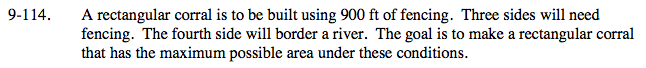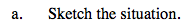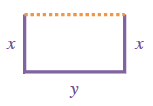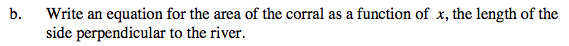2x + y = 900
y = 900 − 2x
Area = (x)(900 − 2x)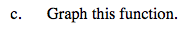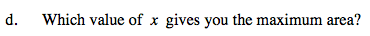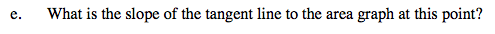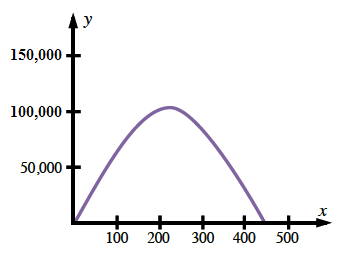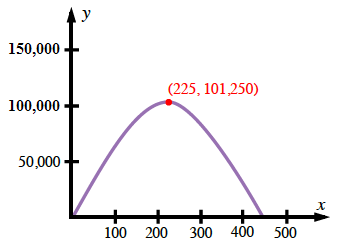Slope of the tangent line is 0.